LIGHT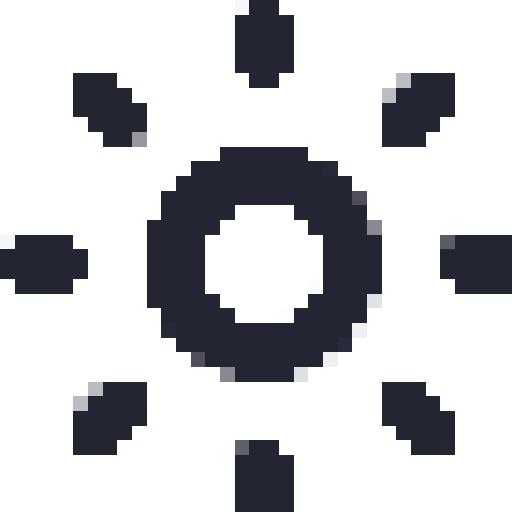DARK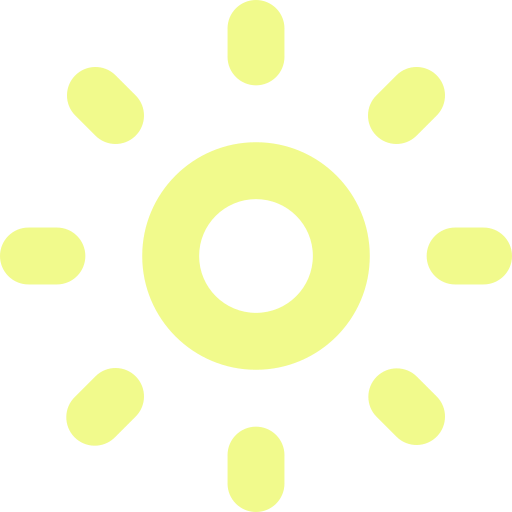BLOG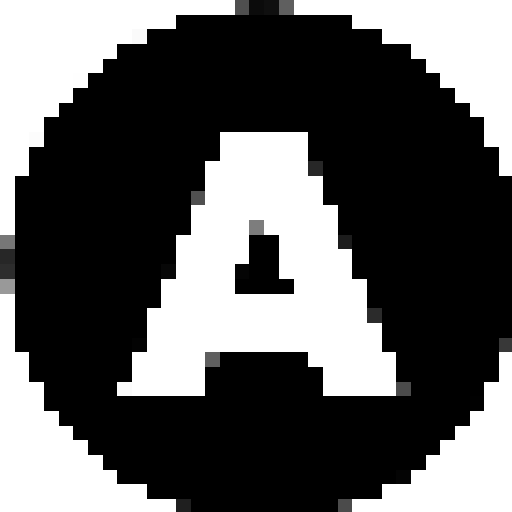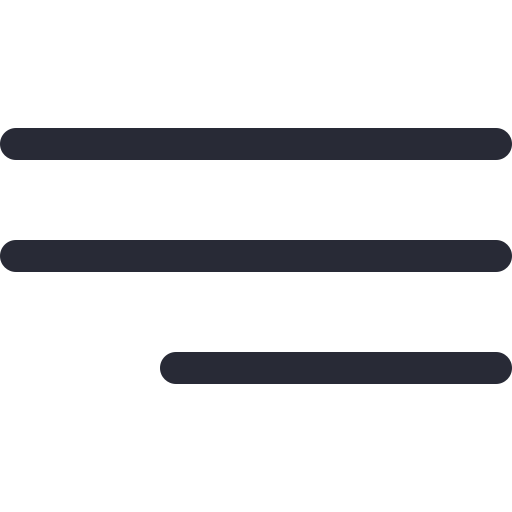# What is tail recursion and why is it useful?

Arjun Aravind 27 July 2021 8 min readRecursion. The old enemy of computer science students in college and high-school; and the destroyer of interest in any subject relating to data structures or algorithms.

Learning to think about a problem in recursive terms can be a daunting task at first but, once you do it for the first time, you'll soon realise that it's a piece of cake. You'll also realise, funnily enough, that it's the easier way to solve certain kinds of problems!

Today, however, we will be focussing on a special variant of a recursive function, the tail recursive function.

To truly grasp why tail recursion is so useful, we will need to first discuss the basics of two concepts:- recursion itself and the computer's call stack. If you're already good with these, feel free to skip!
What is recursion and is it even necessary?
Briefly speaking, recursion is what we call it when a function calls itself in a program.

Any problem that can be broken down into similar but smaller pieces of itself is an example of a problem that can be best solved with recursion.

Examples of this are:-
• Calculating the factorial of a number. The factorial of any number N (except 1) can be re-written as (N * factorial of N-1).
• Traversing a tree structure. Every child node in a tree is a tree itself and it's children are also trees.
• Parsing a JSON object. The value for every key in a JSON object can be a JSON object itself.

Now, can all of these problems be solved only by recursion?

To put it simply, no.

Any problem which has been solved recursively can be solved iteratively (that is, using a loop). And the inverse is true as well, any problem which can be solved iteratively can be solved recursively.

Let's take a simple example. We shall write two functions, both of which calculate the factorial of a given number. The only difference between the functions is that one will be written recursively and one will be written iteratively.

``````/* RECURSIVE FUNCTION */
function recursiveFactorial (givenNumber) {
if (x == 1) return 1;
else return givenNumber * recursiveFactorial (givenNumber-1);
}
``````
``````/* ITERATIVE FUNCTION */
function iterativeFactorial (givenNumber) {
let result = 1;
while (givenNumber > 0) {
result = result * givenNumber;
givenNumber--;
}
return result;
}
``````

Both of these functions do the same thing. But can you see the difference? Even in this simple example, the recursive implementation is much more readable and easier to comprehend.

If you're still not convinced, check out this example!

The point is that using recursion isn't necessary but it's better, when used for certain problems.
• NOTE: Not all problems should be solved recursively though. Before you solve a problem, think first. If the iterative solution is better, than go ahead with that.Break time?
Alright, let's take a deep breath and take a break. Go drink some water. On to the next section!
What does the call stack of a computer do?
Any task that we perform in our day-to-day lives will consist of a set of smaller sub-tasks, which might in-turn consist of even smaller sub-tasks.

When we are doing these smaller tasks, what does our brain remember? It remembers the bigger task, that this sub-task branched off from, so that we can go back to doing that bigger task once we finish doing this sub-task.

Here's an example.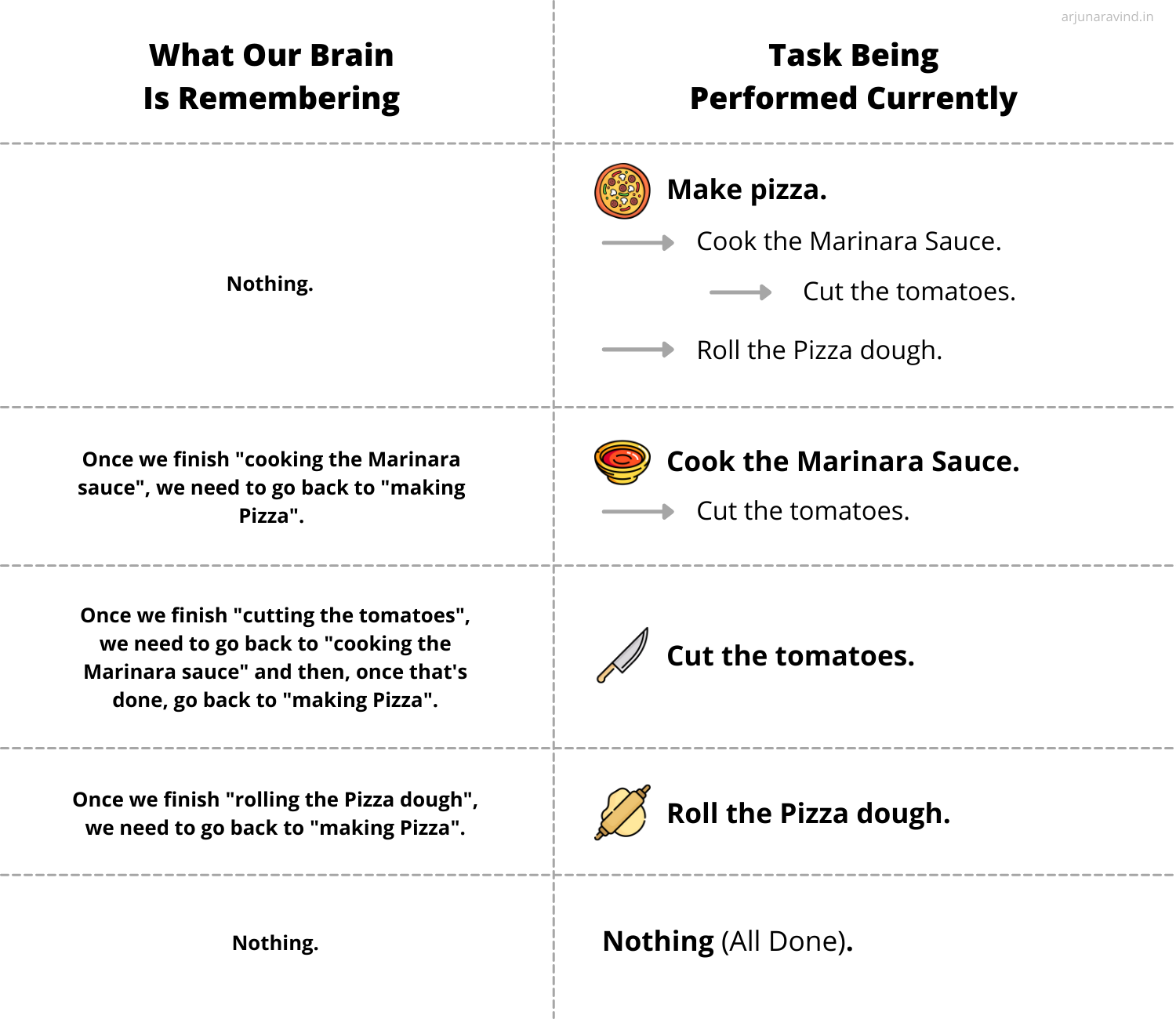I feel like eating some Pizza right now...
The right side shows the tasks and their respective sub-tasks involved in making Pizza. The left side shows what our Brain needs to remember while performing each task.

It's pretty easy to understand, right? When we are cutting vegetables, our brain needs to remember that we are cutting vegetables SO THAT we can cook the sauce SO THAT we can make the pizza.
Similarly, in a program, there will be functions which contain various other function calls, which in-turn, might contain many function calls themselves.
So, just like our brain, when the computer is executing smaller function calls inside a function call, it needs to remember where these function calls originated from so that it can go back to that original function once it's done with these smaller function calls.

Here's the same pizza example but in the form of multiple function calls!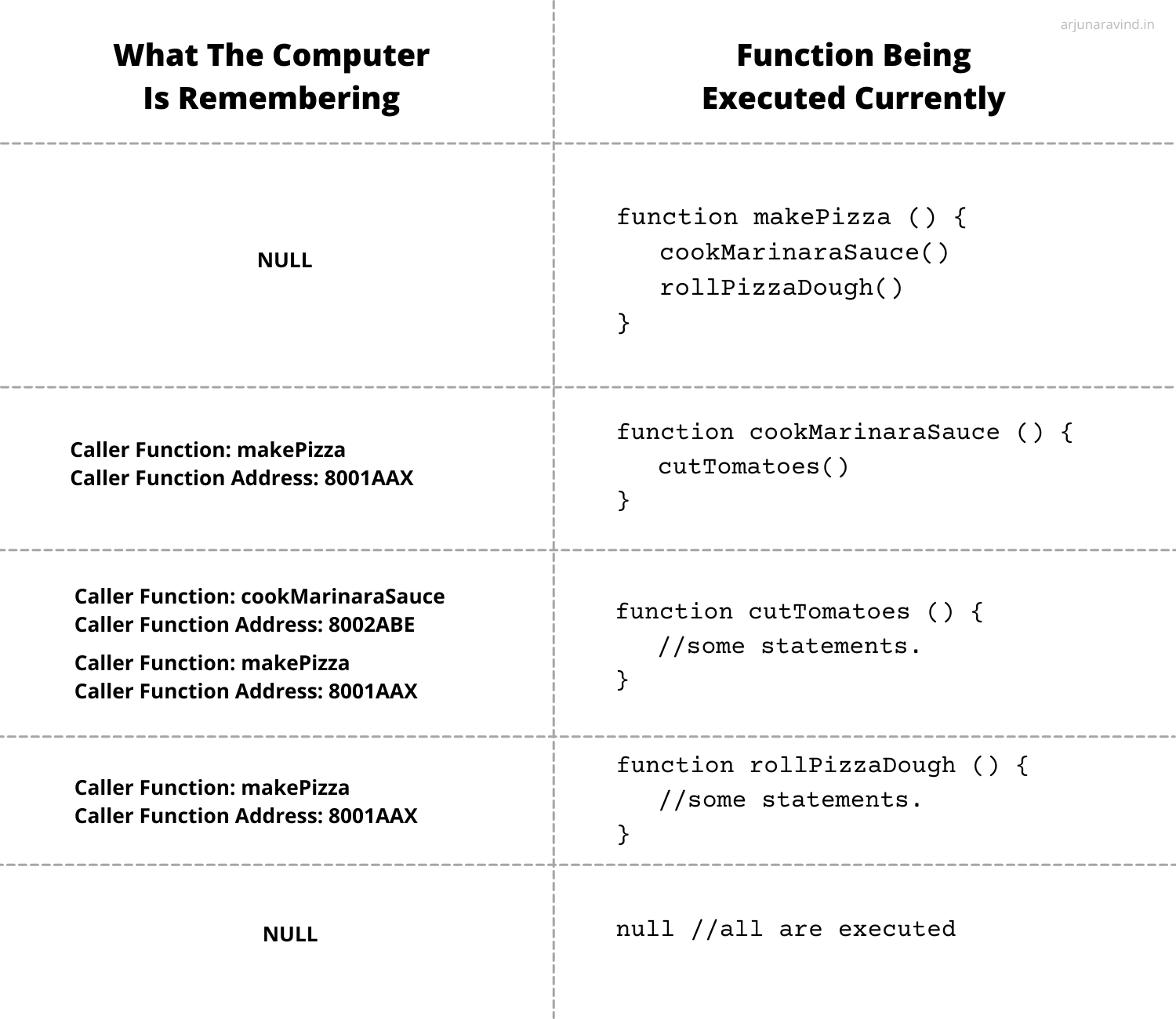This information about function calls and where they originated from is stored by the computer in a placed called the call stack.
Anything else to know about the call stack?
Yes. A call stack is limited by the amount of memory that a computer has. Meaning that if a program has many levels of nested function calls, say in the millions, there is a chance that the call stack may run out of memory and the computer may crash.

You may think, but there can't be any program where the functions might consist of a million levels of nested function calls, right?

Well......there is. Read on!
Introducing Tail Recursion!
We shall start with an example. Let's look at our recursive factorial function from earlier.

``````function recursiveFactorial (givenNumber) {
if (x == 1) return 1;
else return givenNumber * recursiveFactorial (givenNumber-1);
}
``````

Giving an input of 5, let's trace the program.
• recursiveFactorial(5) will return 5 * recursiveFactorial(4)
• recursiveFactorial(4) will return 4 * recursiveFactorial(3)
• recursiveFactorial(3) will return 3 * recursiveFactorial(2)
• recursiveFactorial(2) will return 2 * recursiveFactorial(1)
• recursiveFactorial(1) will return 1

So, recursiveFactorial(5) will not just have to wait for recursiveFactorial(1) to finish, it will have to wait for the value to be returned by recursiveFactorial(2), recursiveFactorial(3) and then recursiveFactorial(4).

Remember the call stack? It has to store all these function calls and track the function which called them (in this case, the same function).

So, for an input of 5, we store 4 function calls in the call stack.

How many function calls are stored when the input is 1,000,000? Do you remember what may happen when there are a very large number of function calls stored in the call stack?
Now, let's try a different approach to the factorial function to try and prevent this from happening.
``````function recursiveFactorial (givenNumber, result) {
if (x == 1) return result;
else return recursiveFactorial (givenNumber-1, result * givenNumber);
}
``````

Notice the difference? We are also passing in the product of result and givenNumber.

Giving an input of 5 for givenNumber and 1 for result, let's trace the program!
• recursiveFactorial(5, 1) will return recursiveFactorial(4, 5)
• recursiveFactorial(4) will return recursiveFactorial(3, 20)
• recursiveFactorial(3) will return recursiveFactorial(2, 60)
• recursiveFactorial(2) will return recursiveFactorial(1, 120)
• recursiveFactorial(1) will return 120

This is amazing! In this case, the factorial is calculated when recursiveFactorial(1) is finished. The value does not need to bubbled up through all those function calls back to recursiveFactorial(5)!

This is what is known as tail recursion. When the recursive function call is the final action of a procedure and the calling function doesn't need to do any further calculation, it is called a tail recursive call.

In our latest example, recursiveFactorial is a tail recursive function. The value of 120 has already been calculated after recursiveFactorial(1).

AND THIS IS WHERE THE MAGIC HAPPENS (in some cases, hehe)!Some language compilers can detect when a function is tail-recursive and prevent the call stack from logging these recursive function calls.

In our case, the computer call stack does not need to keep track of recursiveFactorial(2), recursiveFactorial(3) and recursiveFactorial(4) because they are all going to return the same thing (120) anyway. So, when recursiveFactorial(1) finishes, the program execution immediately jumps back to recursiveFactorial(5).

This could also prevent the call stack from exceeding its memory limit, even if the input number is humungously big!
This optimisation technique is called tail call elimination.
---------------------

Tail recursion is an important part of functional programming languages. Most of the compilers for those languages have some form of tail call optimisation built in.

---------------------

And that's the end of this!

I hope you guys learnt a lot here about the call stack and tail recursion! Feel free to contact me if you have any criticisms, additions or questions. Cheers!